Next: C.3 The Rectangular Potential Up: C Solutions to Schrödinger's Previous: C.1 The Infinite Potential

# C.2 The Finite Potential Well

To show that the infinite well approximation is sufficient in our case, the energy levels of a finite potential well, Fig. C.2, are calculated and compared to (C.10).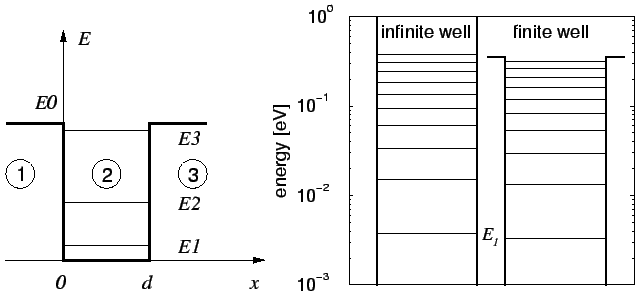The Ansatz for the three regions is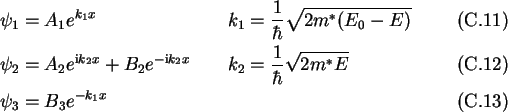The boundary conditions yield the following equations: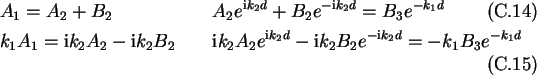Solving for A2 gives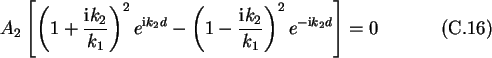Since the trivial solution A2=0 is not of interest, the second term has to be zero. This is the condition fulfilled only for special energies. The transcendent condition can be written as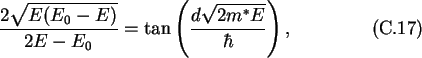which is only numerically solvable. A quantitative comparison is given for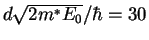, which corresponds to a well with a width of 10 nm and a height of 0.34 eV, in the right side of Fig. C.2. The first energy levels differ only slightly.Next: C.3 The Rectangular Potential Up: C Solutions to Schrödinger's Previous: C.1 The Infinite Potential

Christoph Wasshuber